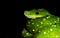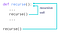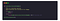# Python Basics: Functions

Complete Intuition With Codes and ExercisePhoto by David Clode on Unsplash

Welcome Guys, To Pythoneers

This Blog Is the Fourth part of the series of Python Basics. In this Blog, We Will Learn about Functions in python. We try to find the answer to what, why, and how.

# What is a Function?

A Function is a set of instructions written outside the main code. A Function is a block of code that only runs when it is called. You can pass data in a function, known as parameters. A Function also have a return type using which it return some value.

In Python, There are two parts to a function. Function Definition and Function Calling. Function Definition is a part of the function which contains the content and expressions of the function. Function Calling is used to call the function so that you can use it in your program.

Function Only Takes Space in the memory when they are called. You Can Define anything inside a function.

# Why Use Functions?

Functions are a set of instruction that is written inside a block of code. There are many reasons to use functions.

1. Code Reusability: Once a Function is Defined it can be used over and over again in our code. You Can Use the same function in your code many times by just invoking it using a function call. Which Saves a lot of time and work for you.
2. Abstraction: Abstraction means hiding the data. In order to use a function, you need to know Four Things.
1. The Name of the function.
2. The Functionality of Function
3. Arguments You Need to give to the function
4. What Kind of result it will return?
Without knowing all of these you can not use the function.

# How To Create a Function?

In Python For Creating Functions, You Need to use the `def` keyword.
Syntax:

`def function_name(attributes):        Task Function Does        -----        -----        return value`

Now Using the above syntax let’s create a function

`def add(num1,num2):    ##Definition ##parameters     sum = num1+num2     return sumadd(5,6) ##calling  ## Arguments`

## Parameter vs Arguments

Parameters are the names used when defining a function and arguments are the those which are passed at the time of calling. Consider a simple example of a function `add()` that adds two numbers and generate their sum. In the function at the time of calling two numbers are passes `add(5,6)` these two numbers are called as arguments and the variable `num1 and num2` which are used in the definition called as Parameter of the function.

# Types of Functions

There are basically two types of functions in python. Inbuild and User Defined.

Build In Functions (Inbuild) Functions are those that comes with the programming language. Every data structure in python have some build in functions.

Some Examples of Build in functions

1. dir() : Returns a list of specified objects
2. eval() : Evaluate and executes and expression
3. len(): Returns the length of the object
4. max(): Find the max from n numbers
5. sum(): Calculates the sum of numbers

User Defined Functions Are Those which are defined by user. The full control of the function is in the hands of the user. user can decide the number of parameters of the function and also the return type of the function. `def` keyword is used to define a user defined functio.

An example of user defined function

`def findmax(a,b):      if a>b:         return a      else:         return bresult = findmax(8,10)print(result)-----------------------------10`

# -: Recursion Function:-

Recursion is a process of defining something in terms of itself in simple words recursion is the process of repeating itself. It can be used as a replacement for the loops in python. A Recursion function is defined as a simple function the difference comes in the calling part. Recursion has two calling statements. one inside the function and another one outside the function.

It can also de defined as a scenario where a function calls itself from its own body.Source

Example of a recursive functionFactorial of a number using Recursive Function
`factorial(5)          # 1st call with 55 * factorial(4)      # 2nd call with 45 * 4 * factorial(3)  # 3rd call with 35 * 4 * 3 * factorial(2) # 4rth call with 2   5 * 4 * 3 * 2 * factorial(1)  # Last call with 1 that returns 1 120                     # Answer`

# -: Anonymous/Lambda Function:-

An anonymous function is a function that is defined without a name. it is also called the lambda function. Lambda function can have any number of arguments but only one expression. The expression inside the lambda function evaluated and returned.

An Example of a simple lambda function

`## Program to add two numbersadd = lambda x,y:x+yprint(add(5,6))----------------------------------11`

Top Writer | ML, DL, Python ,and Programming | Check Out 👉 medium.com/pythoneers

## Sign up for The Weekly Picks

### By Pythoneers

A Newsletter That Contains Top Stories From The Week, Coming On Your Door Every Saturday Take a look.

By signing up, you will create a Medium account if you don’t already have one. Review our Privacy Policy for more information about our privacy practices.

Check your inbox
Medium sent you an email at to complete your subscription.

## More from Pythoneers

Everything Related To Tech And Programming Lives here